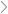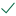Questions & AnswersMYSQLWrite a PROCEDURE called NUM2DIRECTION with one IN parameter of...

QuestionAnswered step-by-step

# Write a PROCEDURE called NUM2DIRECTION with one IN parameter of...

Write a PROCEDURE called NUM2DIRECTION with one IN parameter of type number.

Your procedure will take as input a number between 0 and 360.

It will send to the screen the closest equivalent in letters.

Here are the direction letters for the 16 directions that we will use:

N NNE NE ENE E ESE SE SSE S SSW SW WSW W WNW NW NNW.

We will not use the directions with a "by" in the name.

Below is the correct number mapping for the four main directions. The mapping I posted in the email was wrong.  I misread the figure.

0 = N

90 = E

180 = S

270 = W

You ALSO have to fill in the numbers for the other 12 directions in your procedure.

FOR EXAMPLE:

22.5 = NNE

When you call the procedure and the input is not exactly one of the 16 directions then the procedure has to send the closest letters to the screen.

Thus if the input to the procedure is 22.5 it should send NNE to the screen.

But if the input to the procedure is 20 it should also send NNE to the screen, because NNE is the closest to 20. Closer than N and closer than NE.Solved by verified expert10,000 step-by-step explanations